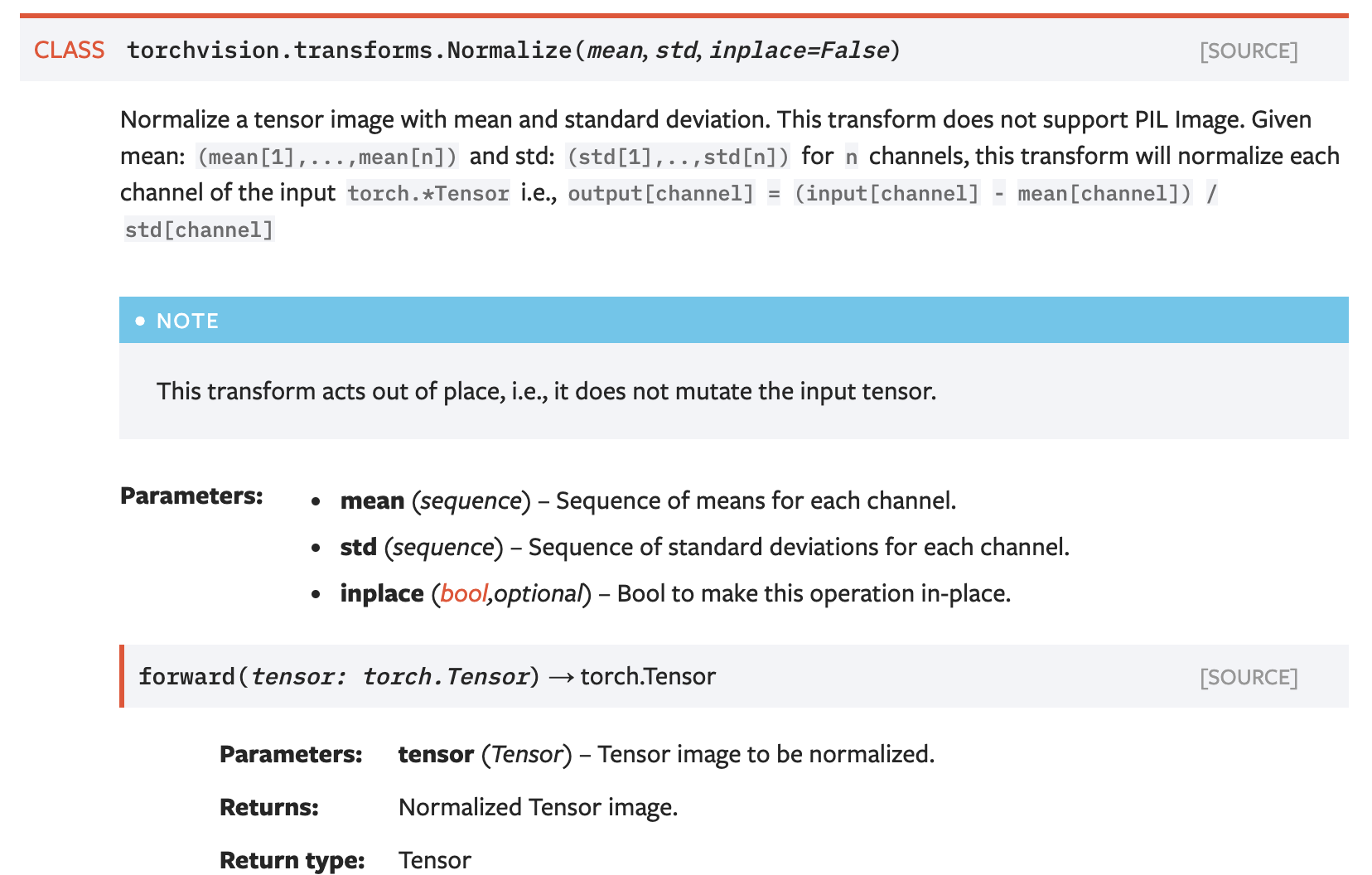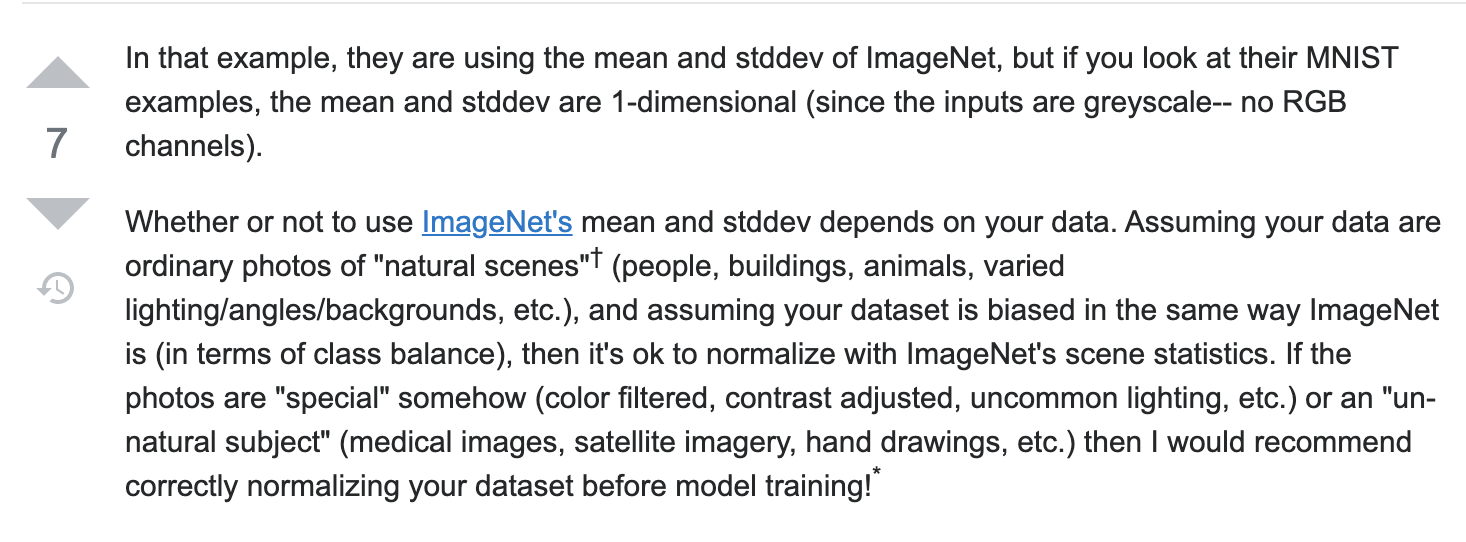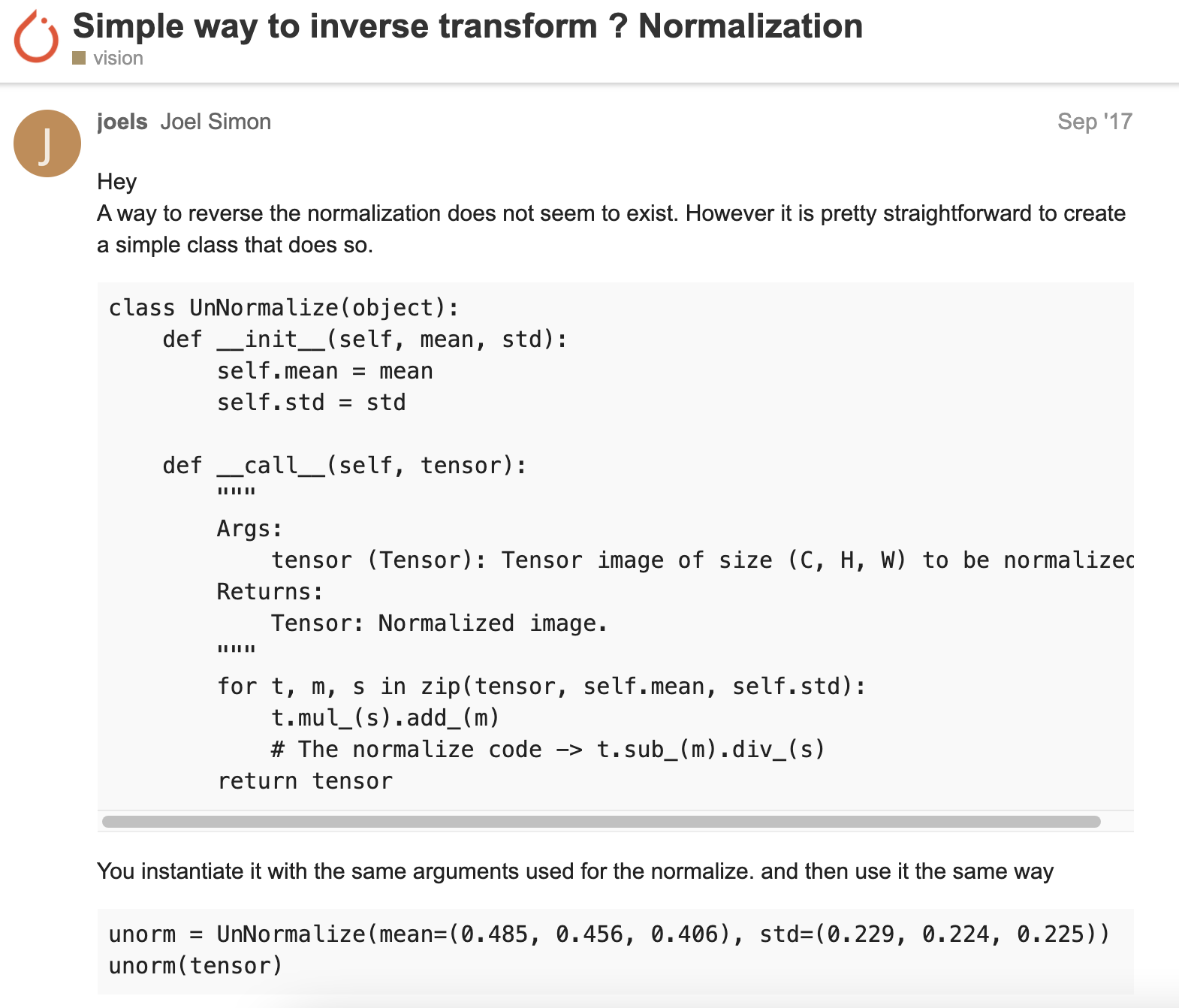• ## PyTorch 数据归一化与反归一化

万次阅读 多人点赞 2019-04-11 11:12:47
文章目录数据归一化除最大值法MinMaxScaler均值和标准差反归一化 数据归一化 除最大值法 def read_and_normalize_train_data(): train_data, train_label = load_train() print('Convert to numpy...') train_...
文章目录数据归一化除最大值法MinMaxScaler均值和标准差反归一化
数据归一化
除最大值法
def read_and_normalize_train_data():

print('Convert to numpy...')
train_data = np.array(train_data, dtype=np.uint8) # now np.amax(train_data)=255

print('Convert to float...')
train_data = train_data.astype('float32')
train_data = train_data / 255
train_target = np_utils.to_categorical(train_target, N_CLASSES)

print('Train shape:', train_data.shape)
print(train_data.shape, 'train samples')
return train_data, train_label

MinMaxScaler
将特征缩放至特定区间   将特征缩放到给定的最小值和最大值之间，或者也可以将每个特征的最大绝对值转换至单位大小。这种方法是对原始数据的线性变换，将数据归一到[0,1]中间。转换函数为:
$x = \frac{x-\min}{\max-\min}$
这种方法有个缺陷就是当有新数据加入时，可能导致max和min的变化，需要重新定义。对于outlier非常敏感，因为outlier影响了max或min值，所以这种方法只适用于数据在一个范围内分布的情况。
无法消除量纲对方差、协方差的影响。
def minmaxscaler(data):
min = np.amin(data)
max = np.amax(data)
return (data - min)/(max-min)

均值和标准差
在分类、聚类算法中，需要使用距离来度量相似性的时候、或者使用PCA技术进行降维的时候，新的数据由于对方差进行了归一化，这时候每个维度的量纲其实已经等价了，每个维度都服从均值为0、方差1的正态分布，在计算距离的时候，每个维度都是去量纲化的，避免了不同量纲的选取对距离计算产生的巨大影响。
def feature_normalize(data):
mu = np.mean(data,axis=0)
std = np.std(data,axis=0)
return (data - mu)/std

pytorch框架下的函数 ：
import torch
import torchvision
import torchvision.transforms as transforms

transform = transforms.Compose(
[transforms.ToTensor(),  # 函数接受PIL Image或numpy.ndarray，将其先由HWC转置为CHW格式，再转为float后每个像素除以255.
transforms.Normalize((0.5, 0.5, 0.5), (0.5, 0.5, 0.5))])

trainset = torchvision.datasets.CIFAR10(root='./data', train=True,
shuffle=True, num_workers=2)

# get some random training images
images, labels = dataiter.next()

上例均值和标准差都是0.5
注意：torchvision.transforms.ToTensor() 函数接受PIL Image或numpy.ndarray，将其先由HWC转置为CHW格式，再转为float后每个像素除以255.
反归一化
def unnormalized_show(img):
img = img * std + mu     # unnormalize
npimg = img.numpy()
plt.figure()
plt.imshow(np.transpose(npimg, (1, 2, 0)))



展开全文pytorch 归一化
• 今天小编就为大家分享一篇pytorch 归一化与反归一化实例，具有很好的参考价值，希望对大家有所帮助。一起跟随小编过来看看吧
• 在建立回归模型时，往往需要对数据进行归一化和反归一化。 然而，一定要注意训练集与测试集使用的归一化参数必须统一！！ 常用的归一化方法有很多，这里以sklearn的MinMaxScale()为例，下面讲述了归一化和反归一化在...
问题描述：
在建立回归模型时，往往需要对数据进行归一化和反归一化。
然而，一定要注意训练集与测试集使用的归一化参数必须统一！！
常用的归一化方法有很多，这里以sklearn的MinMaxScale()为例，下面讲述了归一化和反归一化在建模中的详细过程。
先看一个最普通的归一化例子：
import numpy as np
from sklearn.preprocessing import MinMaxScaler #导入库

data = np.random.randint(0,5,size=5) #随机生成长度为5的数据
m = MinMaxScaler() #建立一个归一化器
data_1 = m.fit_transform(data) #利用m对data进行归一化，并储存data的归一化参数
data_2 = m.inverse_transform(data_1) #利用m对data_1进行反归一化

上面例子只有一组数据data，对data进行归一化，再进行反归一化，流程正确，但在实际建模中几乎不存在这种简单的操作。
因此在正常建模时，对测试集和训练集进行归一化和反归一化时，应执行如下代码：
import pandas as pd
import numpy as np
from sklearn.preprocessing import MinMaxScaler

# 建立数据集:假设训练集有10个样本，测试集有5个样本；两个输入特征，一个输出
train_data = np.random.randint(5,size=(10,3))
test_data = np.random.randint(5,size=(5,3))
train_data = pd.DataFrame(train_data ,columns = ['output','input1','input2'])
test_data = pd.DataFrame(test_data ,columns = ['output','input1','input2'])

# 对训练集和测试集进行归一化
mm = MinMaxScaler()
train_data_m = mm.fit_transform(train_data.values) #注意fit_transform() 和 transform()的区别
test_data_m = mm.transform(test_data.values) #注意fit_transform() 和 transform()的区别

# ...假设这里经过模型训练，得到了模型model;
# 这里model_fit()和model.predict()是假设的模型训练函数和预测函数
model = model_fit(train_data_m) #训练模型
predicted_y_m = model.predict(test_data_m[:,[1,2]]) #利用输入特征input1和input2测试模型
# 这里predicted_y_m.shape为(5,)，例如：array([0.1, 0.2, 0.3, 0.54, 0.5])

# 预测结果反归一化
#先将预测数据设定为前面归一化时设定的维度
predicted_data_m = test_data_m  #将归一化后的test_data_m赋给predicted_data_m
predicted_data_m [:,0] = predicted_y #将第1列的真实值改为预测值
#归一化
predicted_data = mm.inverse_transform(predicted_data_m) #反归一化

# 预测结果为：
predicted_y = predicted_data[:,0]

这里重点需要关注的有两点;
第一点： fit_transform() 和 transform()的区别。两者都是归一化函数，但是fit_transform() 会储存归一化函数是的相关参数，因此对训练集使用fit_transform() ，储存了训练集归一化的相关参数，然后利用这些参数对测试集进行统一的归一化transform()【切记不能再使用fit_transform() ，第二次使用fit_transform() 会刷新mm里储存的参数！！】 。
第二点： 反归一化时任然要使用归一化时储存的参数和格式。归一化时使用的是mm = MinMaxScaler()，因此后面仍然要使用mm进行反归一化；归一化时fit_transform(train_data.values) 中的train_data.values是n3维度（这里10个样本，即为103）的数组，因此反归一化时的数据也必须是3列，即m*3（m为测试集样本数，这里为5）
以上就是以sklearn.preprocessing.MinMaxScaler为例的归一化和反归一化方法。
其他matlab或其他库的归一化和反归一化方法原理大致相同，只要把握以上两点即可。训练集、测试集、归一化、反归一化 均要使用同一参数的归一化函数！！


展开全文• 资源中包括归一化程序及对应的反归一化程序，test程序是对上述两种程序的测试样例。 资源为个人编写，尊重知识产权
• ## python归一化和反归一化

万次阅读 热门讨论 2019-07-17 21:19:17
from sklearn.preprocessing import MinMaxScaler mm = MinMaxScaler() # 归一化 train_label = mm.fit_...# 反归一化 predict_value = mm.inverse_transform(predict_value) 此外，也可以用 from sklearn...
from sklearn.preprocessing import MinMaxScaler

mm = MinMaxScaler()

# 归一化
train_label = mm.fit_transform(train_data)

# 反归一化
predict_value = mm.inverse_transform(predict_value)

此外，也可以用 from sklearn.preprocessing import StandardScaler 进行标准化。

展开全文归一化
• 而在训练过程可视化中，通常需要反归一化。以PyTorch框架而言，提供了torchvision.transforms.Normalize(mean, std, inplace=False)方法用于归一化。 归一化 归一化的实质是将数据的分布根据均值和标准差进行调整。...
前言
在使用深度学习框架构建训练数据时，通常需要数据归一化（Normalize），以利于网络的训练。而在训练过程可视化中，通常需要反归一化。以PyTorch框架而言，提供了torchvision.transforms.Normalize(mean, std, inplace=False)方法用于归一化。归一化
归一化的实质是将数据的分布根据均值和标准差进行调整。
#torchvision.transforms.Normalize(mean, std, inplace=False)
output[channel] = (input[channel] - mean[channel]) / std[channel]

在实际应用过程中通常有三种方式：
（1）普通归一化
将经过ToTensor()方法（能够把范围从[0,255]变换到[0,1]之间）后的数据缩放到[-1, 1]之间。
transforms.Normalize((0.5, 0.5, 0.5), (0.5, 0.5, 0.5))

（2）ImageNet先验归一化
transforms.Normalize((0.485, 0.456, 0.406), (0.229, 0.224, 0.225))

该均值和标准差来源于ImageNet数据集统计得到，如果建立的数据集分布和ImageNet数据集数据分布类似（来自生活真实场景，例如人像、风景、交通工具等），或者使用PyTorch提供的预训练模型，推荐使用该参数归一化。如果建立的数据集并非是生活真实场景（如生物医学图像），则不推荐使用该参数。（3）计算数据集的均值和标准差
对于特定的数据集，可以直接通过对训练集进行统计计算其均值和标准差。
反归一化
针对普通归一化方法的反归一化：
def denorm(x):
out = (x + 1) / 2
return out.clamp_(0, 1)

针对ImageNet先验归一化方法的反归一化：
class UnNormalize(object):
def __init__(self, mean, std):
self.mean = mean
self.std = std

def __call__(self, tensor):
"""
Args:
tensor (Tensor): Tensor image of size (C, H, W) to be normalized.
Returns:
Tensor: Normalized image.
"""
for t, m, s in zip(tensor, self.mean, self.std):
# The normalize code -> t.sub_(m).div_(s)
return tensor

unorm = UnNormalize(mean=(0.485, 0.456, 0.406), std=(0.229, 0.224, 0.225))
unorm(tensor)参考资料
 image processing[数据归一化]均值和方差设置
 torchvision.transforms.Normalize(mean, std, inplace=False)
 How to get these data(means = [0.485, 0.456, 0.406] stds = [0.229, 0.224, 0.225]) in your code? #6
 Why Pytorch officially use mean=[0.485, 0.456, 0.406] and std=[0.229, 0.224, 0.225] to normalize images?
 Simple way to inverse transform ? Normalization
 PyTorch函数之torchvision.transforms.ToTensor()和Normalize()
 pytorch 归一化与反归一化


展开全文人工智能 深度学习 python PyTorch
• 当我们需要对多个指标进行拟合、作图、相干性分析等操作时，如果不同指标之间的量级差距过大会直接影响最终结果，因此我们需要对数据归一化处理，结束后还可以反归一化处理回到真实值。下面介绍matlab中的归一化函数...
• pytorch反归一化 pytorch在进行数据加载时，使用torchvision.transform中的Normalize进行归一化操作，但有时候我们需要在加载之后用到归一化前的数据。 torchvision中，Normalize使用torchvision.transforms....pytorch 深度学习 图像处理
• 验证： 注意：一行一个样本。而mapminmax归一化是按行进行归一化，所以要对...由此可见，用2维的归一化信息，对一维数据进行做反归一化处理，是对1维数据进行了两个反归一化。 k=[1 2;3 4;5 6;9 10]; [k,a] = mapminma深度学习
• 2, 0)) # torch.set_num_threads(3) # img=cv2.cvtColor(img,cv2.COLOR_BGR2RGB) cv2.imshow("sdf", img) cv2.waitKeyEx() 这个测试时间：归一化与反归一化都需要7ms左右， 但是在多路摄像头中，可能比较慢。...
• ## 归一化与反归一化

千次阅读 2020-08-31 17:14:58
1 归一化 归一化就是把需要处理的数据经过处理后（通过某种算法）限制在你需要的一定范围内。 归一化的目的：归一化是为了后面数据处理的方便，其次是提升模型的收敛速度和提升模型的精度。一般可分为以下两种情况 ...神经网络
• matlab归一化和反归一化函数——mapminmax 在做BP神经网络的时候经常会遇到数据的归一化，这个时候需要用到mapminmax函数，老版本可以用premnmx和tramnmx 用函数mapminmax 1 默认的map范围是[-1, 1]，所以如果...matlab 归一化 mapminmax
• ## 数据预处理_数据反归一化01

万次阅读 多人点赞 2019-06-12 15:01:35
数据反归一化 在数据处理中经常用到归一化将数据缩放到一个较为合理的范围。 归一化的方法有很多地方有讲，本篇不做解释 情况1-只对特征进行归一化 将特征和标签，放在相同的数组里，只对特征进行归一化，...norm 归一化
• nnet包实现神经网络看了下网上很多R实现的神经网络都是调用包直接做模型，对于神经网络用来预测的代码根本没有进行归一化和反归一化的求解，在我直接研究了R的scale函数原理后，我先用它对数据归一化，他的反归一化...神经网络 R scale
• 归一化处理我理解，但是很多例子的反归一化我就不是很明白 。如下面这个例子：%准备好训练集%人数(单位：万人)numberOfPeople=[20.55 22.44 25.37 27.13 29.45 30.10 30.96 34.06 36.42 38.09 39.13 39.99 41.93 ...
• 可以加快模型的收敛 output = (input - mean) / std mean:各通道的均值 std：各通道的标准差 图片三个通道 前一个(0.5,0.5,0.5)是设置的mean值 后一个(0.5,0.5,0.5)是是设置各通道的标准差 所以反归一化就是：...
• 问题一：什么是归一化？解答：当数据(x)按照最小值中心化后，再按极差(最大值-最小值)缩放，数据移动了最小值个单位，并且会被收敛到[0，1]之间，而这个过程，就称作数据归一化(Normalization，又称Min-Max Scaling)...
• 2, 0)) # torch.set_num_threads(3) # img=cv2.cvtColor(img,cv2.COLOR_BGR2RGB) cv2.imshow("sdf", img) cv2.waitKeyEx() 这个测试时间：归一化与反归一化都需要7ms左右， 但是在多路摄像头中，可能比较慢。...
•  时空序列预测的任务是通过前N帧格点数据预测后J帧格点数据（类似视频预测，但格点数据最大值不是255...那么在反归一化的时候是使用 (pred*((max(data)-min(data)) + min(data))的方式吗？</p>深度学习...